Courses

# Previous Year Questions: Rotational Motion

## 21 Questions MCQ Test Physics For JEE | Previous Year Questions: Rotational Motion

Description
This mock test of Previous Year Questions: Rotational Motion for JEE helps you for every JEE entrance exam. This contains 21 Multiple Choice Questions for JEE Previous Year Questions: Rotational Motion (mcq) to study with solutions a complete question bank. The solved questions answers in this Previous Year Questions: Rotational Motion quiz give you a good mix of easy questions and tough questions. JEE students definitely take this Previous Year Questions: Rotational Motion exercise for a better result in the exam. You can find other Previous Year Questions: Rotational Motion extra questions, long questions & short questions for JEE on EduRev as well by searching above.
QUESTION: 1

### A solid sphere, a hollow sphere and a ring are released from top of an inclined plane (frictionless) so that they slide down the plane. Then maximum acceleration down the plane is for (no rolling) [AIEEE 2002]

Solution:

As the plane is frictionless, thus there will be only slipping and no rolling. So, for all of them acceleration will be ‘gsinθ’

QUESTION: 2

### Moment of inertia of a circular wire of mass M and radius R about its diameter is [AIEEE 2002]

Solution:

We know that,
MOI through centre (z-axis) is MR², so, by applying perpendicular axis theorem
Ix + Iy = Iz and since Ix = Iy and both are about diameter
Iz = MR², so, 2Ix = MR² MOI about diameter is MR²/2

QUESTION: 3

### A particle of mass m moves along line PC with velocity v as shown. What is the angular momentum of the particle about O ?   [AIEEE 2002]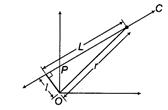Solution:
QUESTION: 4

Initial angular velocity of a circular disc of mass M is w1. Then two small spheres of mass m are attached gently to two diametrically opposite points on the edge of the disc. What is the final angular velocity of the disc ?

[AIEEE 2002]

Solution:
QUESTION: 5

Let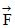be the force acting on a particle having position vector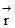and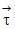be the torque of this force about the origin. Then

[AIEEE 2003]

Solution:
QUESTION: 6

A particle performing uniform circular motion has angular momentum L. If its angular frequency is doubled and its kinetic energy halved, then the new angular momentum is

[AIEEE 2003]

Solution:
QUESTION: 7

A solid sphere is rotating in free space. If the radius of the sphere is increased keeping mass same, which one of the following will not be affected ?

[AIEEE 2004]

Solution:

In free space, neither acceleration due to gravity for external torque act on the rotating solid sphere. Therefore, taking the same mass of sphere if the radius is increased then a moment of inertia, rotational kinetic energy and angular velocity will change but according to the law of conservation of momentum, angular momentum will not change.
In free space, neither acceleration due to gravity for external torque act on the rotating solid sphere. Therefore, taking the same mass of sphere if the radius is increased then a moment of inertia, rotational kinetic energy and angular velocity will change but according to the law of conservation of momentum, angular momentum will not change.

QUESTION: 8

One solid sphere A and another hollow sphere B are of same mass and same outer radii. Their moment of inertia about their diameters are respectively IA and IB such that

[AIEEE 2004]

Solution:

Since the mass density around the axis is greater in solid sphere so the MOI of solid sphere is less for that.

QUESTION: 9

A T shaped object with dimensions shown in the figure, is lying on a smooth floor. A force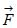is applied at the point P parallel to AB, such that the object has only the translational motion without rotation. Find the location of P with respect to C.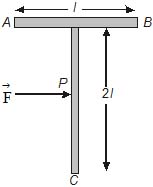[AIEEE 2005]

Solution:

The force F must be applied at COM of this system for it to not produce a torque and hence to avoid rotational motion and have only pure transnational motion. Since same material is used the mass of any portion is proportional to its length. So, the horizontal rod has a mass "m" and the vertical one has mass "2m". Since it is asked to find the position of COM from C let us assume C is the origin (0, 0).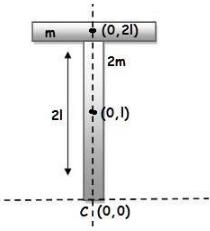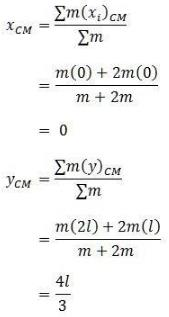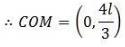So, the position of COM (or) P is at a distance 4l/3 from C

QUESTION: 10

The moment of inertia of uniform semicircular disc of mass M and radius r about a line perpendicular to the plane of the disc through the centre is

[AIEEE 2005]

Solution: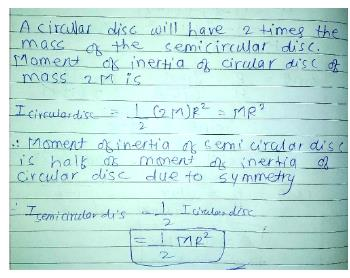QUESTION: 11

An angular ring with inner and outer radii R1 and R2 is rolling without slipping with a uniform angular speed. The ratio of the forces experienced by the two particles situated on the inner and outer parts of the ring,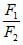is

[AIEEE 2005]

Solution:
QUESTION: 12

A thin circular ring of mass m and radius R is rotating about its axis with a constant angular velocity w. Two objects each of mass M are attached gently to the opposite ends of a diameter of the ring. The ring now rotates with an angular velocity w' =

[AIEEE 2006]

Solution:

The initial angular momentum of the ring will be:
Li​=mR2ω
whereas the final angular momentum is given as:
Lf​=mR2ωnew​+2MR2ωnew
Conserving angular momentum
mR2ω=(mR2+2MR2new​
⇒ωnew​=m​ω/m+2M

QUESTION: 13

Four point masses, each of value m, are placed at the corners of a square ABCD of side l. The moment of inertia of this system about an axis passing through A and parallel to BD is

[AIEEE 2006]

Solution:
QUESTION: 14

Angular momentum of the particle rotating with a central force is constant due to

[AIEEE 2007]

Solution:

By law of conservation of angular momentum, in the absence of external torque angular momentum is conserved.
For angular momentum to be constant
τ = 0
Central force: zero torque

QUESTION: 15

A round uniform body of radius R, mass M and moment of inertia I, rolls down (without slipping) an inclined plane making an angle q with the horizontal. Then its acceleration is

[AIEEE 2007]

Solution: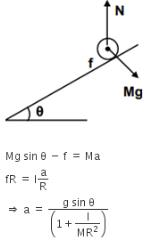QUESTION: 16

For the given uniform square lamina ABCD, whose centre is O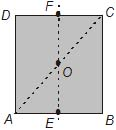[AIEEE 2007]

Solution: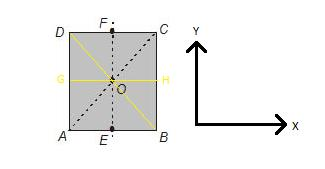By symmetry we can say that
IEF = IGH
IAC = IBD
By perpendicular axis theorem, we can say that
IZ = IEF + IGH = 2IEF
Also, IZ = IAC + IBD = 2IAC
Hence, IAC = IEF

QUESTION: 17

Consider a uniform square plate of side a and mass m. The moment of inertia of this plate about an axis perpendicular to its plane and passing through one of its corners is

[AIEEE 2008]

Solution:

Icm = ma2/6
Now, applying parallel axis theorem
Ireq = Icm + m(a/√2)2
Ireq = 2/3 ma2

QUESTION: 18

A thin uniform rod of length l and mass m is swinging freely about a horizontal axis passing through its end. Its maximum angular speed is w. Its centre of mass rises to maximum height of

[AIEEE 2009]

Solution:

The uniform rod of length l and mass m is swinging about an axis passing through the end. When the centre of mass is raised through h, the increase in potential energy is mgh. This is equal to the kinetic energy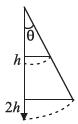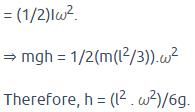QUESTION: 19

A pulley of radius 2 m is rotated about its axis by a force F = (20t _ 5t2) N (where t is measured in seconds) applied tangentially. It the moment of inertia of the pulley about its axis of rotation is 10 kg-m2 the number of rotations made by the pulley before its direction of motion if reserved, is

[AIEEE 2011]

Solution:
QUESTION: 20

A thin horizontal circular disc is rotating about a vertical axis passing through its centre. An insect is at rest at a point near the rim of the disc. The insect now moves along a diameter of the disc to reach its other end. During the journey of the insect, the angular speed of the disc.

[AIEEE 2011]

Solution:

Angular momentum L=Iω
L=mr2×ω
Since r first decreases then increases.
So due to conservation of angular momentum L first increases then decreases.

QUESTION: 21

A hoop of radius r and mass m rotating with an angular velocity w0 is placed on a rough horizontal surface. The initial velocity of the centre of the hoop is zero. What will be the velocity of the centre of the hoop when it cases to slip ?

[JEE Mains 2013]

Solution: